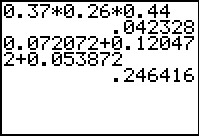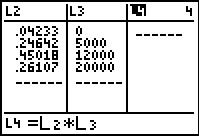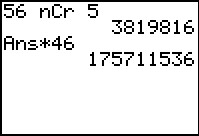# Activities

••• ##### Subject Area

• Math: Statistics: Normal Distributions
• Math: Statistics: Probability and Random Variables

• ##### Author9-12

20 Minutes

• ##### Device
• TI-83 Plus Family
• TI-84 Plus
• TI-84 Plus Silver Edition
•TI-84 Plus C Silver Edition
•TI-84 Plus CE
• ##### Report an Issue

It's To Be Expected#### Activity Overview

Students use a tree diagram to find theoretical probabilities and use this information with lists to find the expected value.

#### Key Steps

•Students will be introduced to expected value. They will use lists to calculate the expected value of the contest, given that each number of baskets is associated with a different dollar amount.

•Students will use a diagram to model three basketball players making a shot for a charity contest. Using the diagram as a guide, they will calculate the probabilities of each branch. Then students will add up the necessary probabilities to determine the overall probability of none, one, two, or all of the players making their shot.

•The extension is an application of expected value. Students will find the expected value for a given jackpot of a lottery that requires the winning ticket to match six numbers and, given the cost of a ticket, students find the lottery operator’s break-even point.